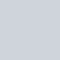# 先來看看今天的結果圖## -> 此篇文章的程式碼 github

Day18_圖片二值化_threshold.ipynb

# 運用 OpenCV 做圖片二值化，產生黑白的圖片

``````img = cv2.cvtColor(img, cv2.COLOR_BGR2GRAY) # 先將圖片轉為灰階

# 將小於閾值的灰度值設為0，其他值設為最大灰度值。>127 =255, <127 =0
ret,thresh1 = cv2.threshold(img,127,255,cv2.THRESH_BINARY)

# 將大於閾值的灰度值設為0，其他值設為最大灰度值。>127 =0, <127 =255
ret,thresh2 = cv2.threshold(img,127,255,cv2.THRESH_BINARY_INV)

# 將大於閾值的灰度值設為閾值，小於閾值的值保持不變。 >127 =127
ret,thresh3 = cv2.threshold(img,127,255,cv2.THRESH_TRUNC)

# 將小於閾值的灰度值設為0，大於閾值的值保持不變。 <127 =0
ret,thresh4 = cv2.threshold(img,127,255,cv2.THRESH_TOZERO)

# 將大於閾值的灰度值設為0，小於閾值的值保持不變。 >127 =0
ret,thresh5 = cv2.threshold(img,127,255,cv2.THRESH_TOZERO_INV)
``````

`cv2.threshold` 最主要的功能是能夠幫助我們將一張圖片做二值化，

``````cv2.threshold(img, 閥值, 最大灰度值, 使用的二值化方法)
``````

(假設`閥值=127、最大灰度值=255`)

• cv2.THRESH_BINARY
• 將小於閾值的灰度值設為0，其他值設為最大灰度值。
• 白話文：`>127 =255, <127 =0`
• cv2.THRESH_BINARY_INV
• 將大於閾值的灰度值設為0，其他值設為最大灰度值。
• 白話文：`>127 =0, <127 =255`
• cv2.THRESH_TRUNC
• 將大於閾值的灰度值設為閾值，小於閾值的值保持不變。
• 白話文：`>127 =127`
• cv2.THRESH_TOZERO
• 將小於閾值的灰度值設為0，大於閾值的值保持不變。
• 白話文：`<127 =0`
• cv2.THRESH_TOZERO_INV
• 將大於閾值的灰度值設為0，小於閾值的值保持不變。
• 白話文：`>127 =0`# 為什麼我們需要做二值化呢?

【沒錢ps,我用OpenCV!】Day 18 – 進階修圖5，運用 OpenCV 做圖片二值化，產生黑白的圖片吧！cv2.threshold 各種選擇參數大全

# Reference

https://blog.csdn.net/on2way/article/details/46812121